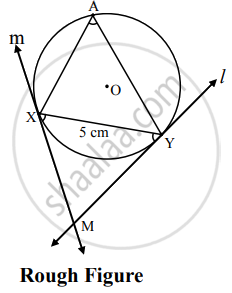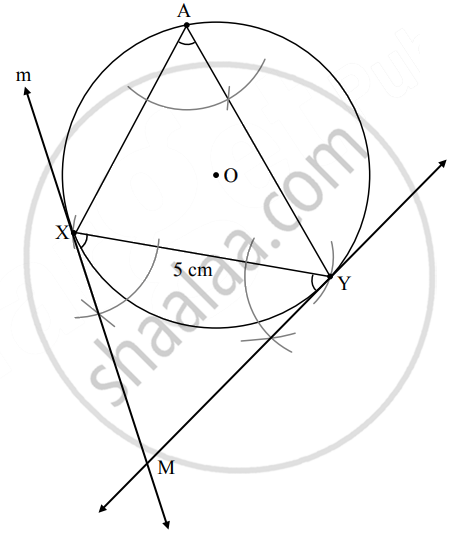# Draw a circle of radius 3 cm and draw chord XY 5 cm long. Draw the tangent of the circle passing through point X and point Y (without using the center of the circle) - Geometry

Diagram

Draw a circle of radius 3 cm and draw chord XY 5 cm long. Draw the tangent of the circle passing through point X and point Y (without using the center of the circle)

#### SolutionAnalysis:

As shown in the figure, line l, m are the tangents to the circle at points Y, X respectively.

seg XY is a chord of the circle and ∠XAY is an inscribed angle. By tangent secant angle theorem,

∠XAY = ∠YXM and ∠XAY = ∠XYM

By converse of tangent secant angle theorem,

If we draw ∠XYM such that ∠XAY = ∠XYM, then ray YM

i.e. (line l) is a tangent at point Y.

Also, if we draw ∠YXM such that ∠XAY = ∠YXM, then ray XM.

i.e. (line m) is a tangent at point X.Steps of construction:

1. Draw a circle of radius 3 cm.
2. Draw a chord XY of length 5 cm.
3. Take a point A on the major arc, other than X and Y.
4. Join XA and YA.
5. Using X and Y as vertices and chord XY as one side, draw ∠XYM and ∠YXM equal to ∠XAY.
6. Lines containing the rays XM and YM are the tangents to the circle at X and Y respectively.
Concept: Construction of a Tangent to the Circle at a Point on the Circle
Is there an error in this question or solution?

Share